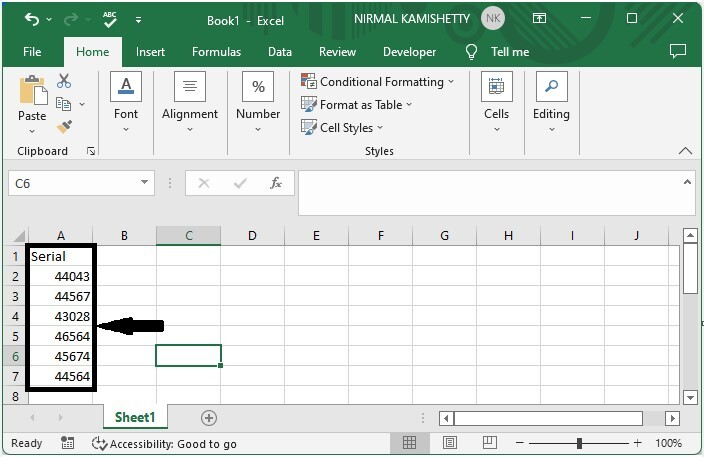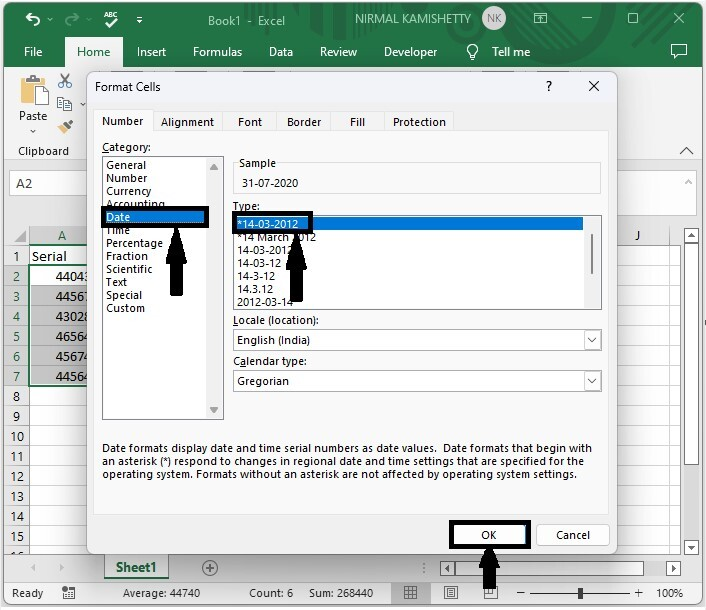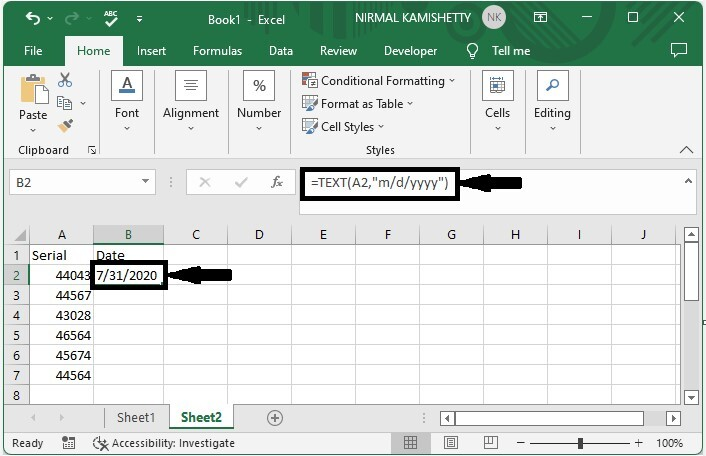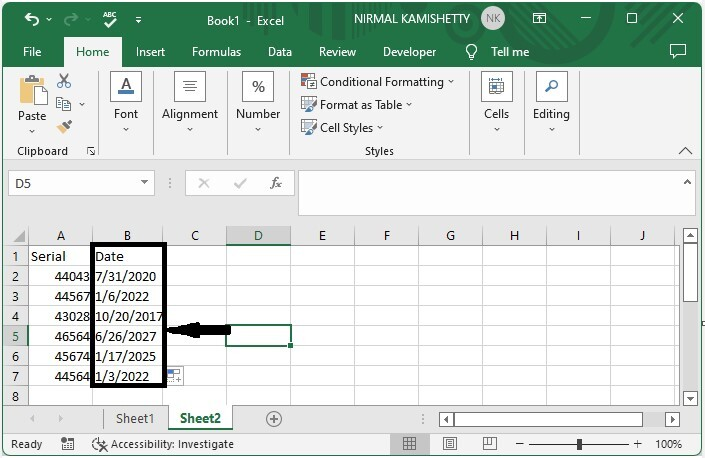# How to Convert Serial Number to Date in Excel?

When you enter dates into an Excel sheet, you may notice that the date is displayed as a number. So, we need to convert them into dates. In this article, we will learn how to convert a serial number to a date in Excel. We can solve this problem by using two methods: the first is by using the format, and the second is by using the formulas supported by Excel.

In Excel, every row has a serial number connected to it. Here we will use two methods to complete the task: the first is by using the format function, and the other is by using the formula.

## Converting Serial Number to Date Using Format

Here, we will first select the data and then use the format to complete the task. Let us look at a simple procedure for converting a serial number to a date in Excel using a format.

### Step 1

Let us consider an Excel sheet where the data in the sheet is similar to the data shown in the below image.Now select the data, then right-click and select format cells; in the popup, click on date, choose format, and click OK.

Select data > Right click > Format cells > Date > Format > OK/## Converting Serial Number to Date Using Formulas

Here, we will first use the TEXT formula to get any one of the results, then use the auto-fill handle to get all the results. Let's go over a simple procedure for converting a serial number to a date in Excel using formulas.

### Step 1

Let us consider the same data that we used in the above example.

Now click on the empty cell in our cell B2 and enter the formula as =TEXT(A2,"m/d/yyyy") and click enter to get our first result. In the formula, A2 is the address of the cell value in the sheet.

Empty cell > Enter formula > Enter### Step 2

To get all the results, drag down from the first result using the auto-fill handle, and our final result will be similar to the below image.## Conclusion

In this tutorial, we used a simple example to demonstrate how you can convert a serial number to a date in Excel.

Updated on: 06-Mar-2023

185 Views